## Conditional probability: why is it defined like that?

So, you want to calculate the probability of an event knowing that another has happened. There is a formula for that, it is called conditional probability, but why is it the way it is? Let’s first write down the definition of conditional probability: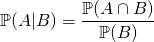We need to wonder: what does the happening of eventtell about the odds of happening of event? How much more likelybecomes ifhappens? Think in terms of howaffects.

Ifandare independent, then knowing something about B will not tell us anything at all about, at least not that we did not know already. In this case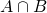is empty and thus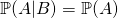. This makes sense! In fact, consider this example: how does me buying a copybook affects the likelihood that your grandma is going to buy a frying pan? It does not: the first event has no influence on the second, thus the conditional probability is just the same as the normal probability of the first event.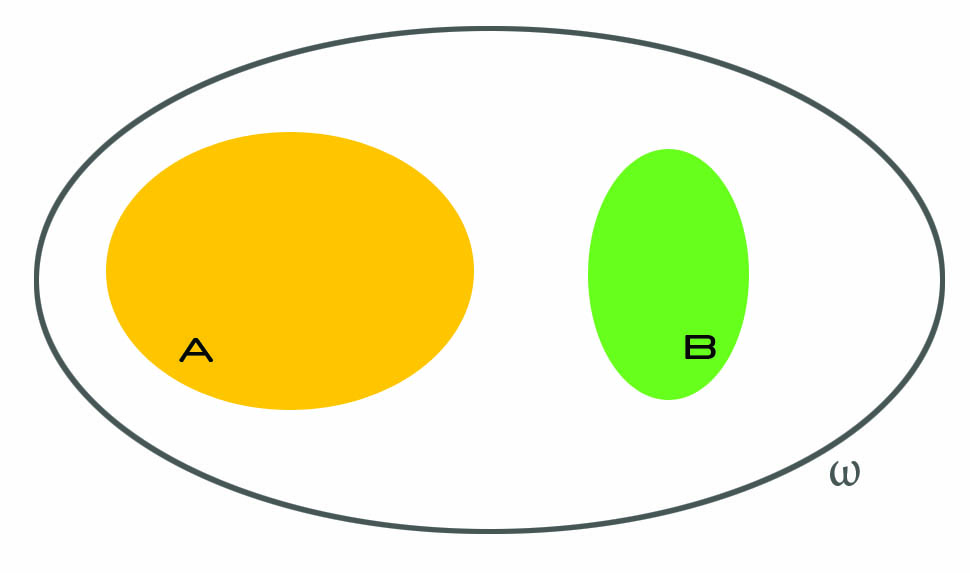Ifandare not independent, several things can happen, and that is where things get interesting. We know that B happened, and we should now think as ifwas our whole universe. The idea is: we already know what are the odds of, right? It is just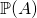. But how do they increase if we know that we do not really have to consider all possible events, but just a subset of them? As an example, think ofversusknowing that all balls are red. This makes a huge difference, right? (As an aside, that is what we mean when we say that probability is a measure of our ignorance.)

So anyway, now we ask: what is the probability of? Well, it would just be, but we must account for the fact that we now live inside, and everything that is outside it is as if it did not existed. Soactually becomes: we only care about the part ofthat is inside, because that is where we live now.

But, there is a caveat. Continue reading “Conditional probability: why is it defined like that?”